# Validation of a simplified method for the evaluation of pressure and temperature on a RR Merlin XX head

Validation of a simplified method for the evaluation of pressure and temperature on a RR Merlin XX head

Luca Piancastelli*A. Burnelli Stefano Cassani

Department of Industrial Engineering, Alma Mater Studiorum University of Bologna, Viale Risorgimento, 2 - Bologna (BO), Italy

MultiProjecta, Via Casola Canina, Imola (BO), Italy

Corresponding Author Email:
luca.piancastelli@unibo.it
Page:
549-558
|
DOI:
https://doi.org/10.18280/ijht.350311
|
Accepted:
|
Published:
30 September 2017
| Citation

OPEN ACCESS

Abstract:

Material behavior depends on average peak temperature, stress magnitude and stress gradient. This assumption is valid since temperatures varies slowly when compared to pressure (stress). In this paper, a RR Merlin head is simulated with a few mathematical models used in Formula 1 racing. These extremely simplified models make it possible to evaluate temperatures and pressures starting from very few data. The method is described in detail, along with the many experimental coefficients available from several years of design activity. A step by step approach is used to allow the comprehension of this method that was developed by the Authors. The choice of the RR Merlin was dictated by the public availability of experimental data on temperatures. In fact, in the case of the RR Merlin XX, very reliable experimental results are available in NACA TN 2069. A reverse engineering process was applied on a rescued RR Merlin XX head. An accurate redesign was performed to obtain a 3D model. Assembly instructions and tolerances were found on original Rolls Royce overhaul manuals. In this way assembly and working loads were calculated and simulated. Nonlinear FEA analysis was performed on this CAD model with extremely satisfactory results for the thermal loads. Well known criticalities of the original design were found. The results were compared with NACA results both for heat rejection and temperatures. However, the mechanical stresses proved to be more critical for simulation and evaluation. Therefore, they will be discussed in another, dedicated paper.

Keywords:

optimization, simulation, CAD, geometry, FEA, thermal analysis, piston engine

1. Introduction

A significant portion of the structural loads acting on the head during operation is derived from the thermal field. The local temperature distribution slightly affects cylinder filling and cylinder gas temperatures. In fact, "modest" wall temperature differences are negligible in performance, as the gas-to-wall temperature gap is very high . An overall increase in wall temperatures slightly penalizes engine performance in terms of volumetric efficiency: the density of the intake charge is reduced while tending to decrease the fraction of heat dissipated by the walls during combustion. It increases the maximum cycle temperature and improves thermodynamic performance. In this way, it increases also the amount of energy actually available to be converted. Between the two effects (reduction of volumetric efficiency and increase of thermodynamic efficiency), the first one shows that a rise in temperature near the intake ducts of the order of 30% (from 393K to 453K) leads to a reduction in power in the order of a modest "7-8%. However, another fundamental aspect is that the head temperature greatly affects the mechanical properties of the material and the degradation of the lubricant. Aluminum alloy strength, for example, drops rapidly beyond 473 K and at 573 K (the strength) is reduced by more than 50%. Furthermore, lubricant is oxidized more and more rapidly as the temperature rises. An engine can operate under "constant" (or steady-state or stationary) conditions of temperature, “constant” load and “constant” velocity or under "transient" conditions, when it is necessary to switch from one functioning point to another. An engine mounted on a car or a motorcycle never works under stationary conditions and therefore, it would be necessary to simulate different operating conditions, either stationary or transient, by checking one by one . However, since the time to devote to design is limited and the goal is always to get in production as soon as possible, during design it is convenient to test the head under the maximum power and temperature conditions, which in many respects are the most unfavorable. Any checks at maximum and transient torque can be performed on the final prototypes before they are sent to production, as they take longer to execute and to interpret the results . Since the thermo-structural verification takes place in stationary, high-power operating conditions, some considerations can be made:

- the geometry of the system continuously changes with the movement of the piston;

- Even if the engine operates under stationary conditions, there are cyclical transformations inside with fluid motion that is never identical to itself in passing from one cycle to another;

- the temperature and the speed of the gases assume different values ​​from point to point during the cycle;

- the thermal conductivity of the wall depends on the amount of lubricant, fuel and deposits on it;

- fluid motion conditions exert an important influence on heat exchange with walls, pollutant formation and engine performance;

- several studies show that the variations in the time of thermal flux occur at a rapid rate that causes very small variations in temperature on the surface of the combustion chamber, which are safely negligible for the purpose of structural analysis;

- a negligible fraction of the heat transmitted to the liner of the cylinder is caused by friction with the piston.

Temperature variations in the temperature can be distinguished in short and long-term variations: the long-term response (in the order of seconds) is that related to the load variations, the short-term result derives from the fluctuations in pressure and temperature of gases during the cycle, with response in milliseconds .  In this paper, we will focus on the steady state, high load design point.

## 1.png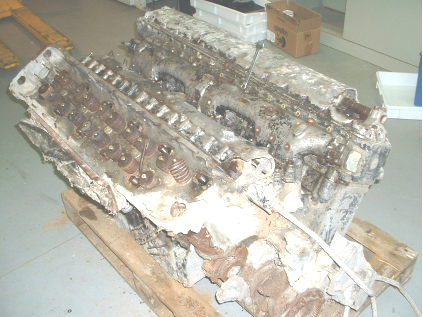Figure 1. The rescued engine before the restoration

2. Historical Notes

On November 20, 1943, at 0226 Italian time, a De Havilland Mosquito DH.98 FB Mk VI of RAF 23th Squadron crashed on fire in the natural park of San Marino, just below the Montalto house, that is on the top of a small hill. The crew composed by F/Sgt (1213930) Donald Hepworth BENTLEY (pilot) RAFVR and Sgt (1323213) Victor Horace CAUSEWAY (nav.) RAFVR – were killed instantly. One of the engines of this aircraft laid in a depot up to 2005. The engine, damaged in the crash and widely savaged through the years, was rescued by Carlo Bezziccheri of the University of Bologna and brought to the Bologna University Site of Forli’ for (partial) restoration and studies (Fig. 1,2,3). Then it was given back to the owner, the Aero Club of San Marino. This RR Merlin XX engine had been widely studied by the Authors.  The Authors wish to thank the Aero Club of San Marino, Carlo Bezziccheri and Giuseppe Macina for the courtesy. The engine plate is missing, but with any probability the engine is the left (port) one. This paper comes from this activity of reverse engineering, conceived to determine the design parameters and criteria for aircraft piston engines. The Rolls Royce Merlin is ideal for this purpose. In fact, it was developed fully during WWII and several data are available from bibliography. Unfortunately, the original design criteria and methods were not entirely published by the Rolls Royce engineers. Therefore, this reverse engineering work is necessary for future aircraft piston engine design.

## 2.png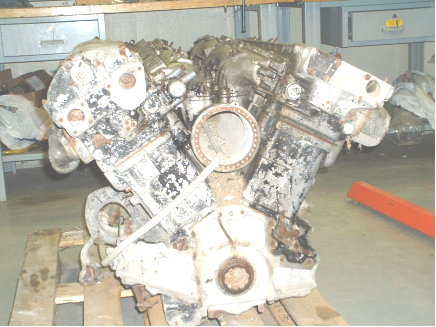Figure 2. The rescued engine

The engine appears heavily damaged mainly by the original crash. The right head (from behind) is missing of a part of the casting and of the crankshaft.

## 3.png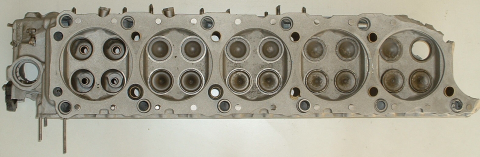3. General Considerations

Starting from the assumption to study a stationary engine operating condition, it will be shown that it is sufficient to refer to temperature-average values over time, thus to a one-dimensional approach. The assumption of a one-dimensional model is justified by the reduced depth of penetration of the variable temperature during the cycle. Temperature variations can be calculated with the Fourier’s equation (1).

$\rho c \frac{\partial T}{\partial t}=\lambda \frac{\partial}{\partial x}\left(\frac{\partial T}{\partial x}\right)+q$    (1)

where q is the heat generated inside the material. In our case, equation (1) can be rewritten in equation (2).

$\frac{\partial T}{\partial t}=\alpha^{2} \frac{\partial^{2} T}{\partial x^{2}}$  (2)

where α2=λ/ρ c. To separate the variables, we suppose that the function of the temperature is given by the product of two functions: $T(x, t)=X(x) \theta(t)$ . Therefore, equation (2) can be rewritten into equation (3).

$\frac{1}{\alpha^{2} \theta} \frac{d \theta}{d t}=\frac{1}{X} \frac{d^{2} X}{d x^{2}}$     (3)

Since the first member is function only of t and the second only function of x, the previous equation can be replaced with two linear equations of ordinary derivatives (4) and (5)

$\frac{1}{\theta} \frac{d \theta}{d t}=-\alpha^{2} a^{2}$    (4)

$\frac{1}{X} \frac{d^{2} X}{d x^{2}}=-a^{2}$     (5)

Whose integrals are respectively equations (6) and (7) and a particular integral of (1) is equation (8).

$\theta(t)=A e^{-\alpha^{2} a^{2} t}$     (6)

$X(x)=B \operatorname{sen}(\alpha x)+C \cos (a x)$      (7)

$T=\left[A_{1} \operatorname{sen}(\alpha x)+A_{2} \cos (a x)\right] e^{-\alpha^{2} a^{2} t}$     (8)

Now we have to fix the initial and boundary conditions.

Let's suppose that the temperature at first is uniform and equal to T0; we also assume that at infinite distance the temperature remains constant, whereas for x = 0 varies with a law that we approximate with a sinusoidal function of the type (9):

$T(0, t)=T_{0}+\Delta T_{0} \operatorname{sen} \omega t$    (9)

It can be verified that the integral becomes (10):

$T(x, t)=T_{0}+\Delta T_{0} e^{-m x} \operatorname{sen}(\omega t-m x)$     (10)

With (11)

$m=\sqrt{\omega \rho c / 2 \lambda}$      (11)

Therefore, the temperature oscillations that are on the surface are damped inward with an exponential factor that is the function of the material, the distance from the surface and the pulsation frequency.

Considering a Merlin 4-stroke engine, which rotates at 3000 rpm (fc=25), the angular velocity ω of (11) is (12):

$\omega=2 \pi f_{c}=\frac{2 \pi 3000}{120} \approx 157$     (12)

The distance $\overline{x}$ at which the oscillation amplitude is 1/100th of the surface one is (13-14):

$\Delta T_{0} e^{-n \pi}=\Delta T_{0} / 100$      (13)

$-m \overline{x}=-\ln 100$        (14)

With an aluminum alloy the average values for (11) are

$\rho=2700\left[\mathrm{kg} / \mathrm{m}^{3}\right] \quad c=930[\mathrm{J} / \mathrm{kg} K] \quad \lambda=220[\mathrm{W} / \mathrm{m} \mathrm{K}]$

Therefore $\overline{x}$ is 4.8 mm. For the reference value ΔT0, a reasonable value for an engine running at 3000rpm is 4 DEG C.  The percentage attenuation of T0 with $\overline{x}$ is shown in Figure 1.

## 0001.png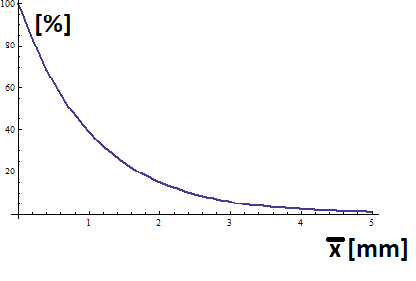Figure 1. Attenuation of temperature with distance from the surface (aluminum alloy)

From Figure 1 it is possible to see that the temperature oscillation in an aluminum alloy at a depth of 1mm are negligible. Therefore, time averaged values can be used for the simulation.  Numerous studies have shown that the temperature and heat flow to which the combustion chamber is subjected varies considerably, due to the flame path, the gas movement, and the cooling passages. It is not always true that the area adjacent to the intake valves is cooler than the one near the exhaust valves, in spite of the fact that intake valves enjoys additional cooling during the supply of fresh gas while the exhaust valves receives heat during discharge.

Also, even if the chamber and the cooling ducts are perfectly symmetrical, due to the gas and flame front movement, it is normal to find temperature differences that can be measured with thermocouples positioned near the wall of the combustion chamber as shown in NACA TN 2069.

NACA TN 2069 is about “Cylinder head temperatures and coolant head rejection of a multicylinder liquid cooled engine of 1650 cc displacement”. This engine is the Merlin Packard V-1650-7 engine which is a slightly improved copy of our Merlin XX engine. The presence of deposits constitutes a further variable since they act as an insulating layer. The valves are another complication of the problem, as they exchange heat with the gases and through contact with seats and guides with the head. A model that takes into account the differences in temperature and heat flow in the various areas of the head is therefore indispensable. With a reasonable approximation, the average thermal flow at one point of the head can be determined, considering the total thermal flow divided into several areas, each with its own coefficient.

A simplified approach of this approximation is based on the fact that, as you proceed from the cylinder axys toward the liner, the thermal flow decreases to about half the one of the central zone. By applying this simplified model, you can only consider the distance (r) from the center of the combustion chamber as the only parameter. In this case the parabolic relationship of equation (15) is the most common:

HeatFlux$_{\text {Fraction}}=1-0.5\left[\frac{r}{R_{0}}\right]^{2}$        (15)

where R0 is the cylinder radius (bore/2).  The properties of the fluid operating the thermodynamic cycle vary in a negligible manner during the cycle. Referring to the case of the Merlin engine, a two-phase, liquid-gas, mixture of fuel (gasoline-AVGAS) and comburent (air) is aspirated.

Normally, the carburetor or the injection system provides a mixture whose air-to-gas ratio is between 12 and 18 (in mass). The designer provides all the possible options for a complete vaporization and good fuel mixing to ensure a good engine operation. When the engine is working steady-state, gasoline, which is injected in liquid form, is completely vaporized downstream of the intake valves. Therefore, it is necessary to take into account the two-phase fluid nature and the heat subtraction by the vaporization of the fuel only in the intake duct. However, for the purpose of fluid motion in ducts and drainage coefficients, it turns out that the properties of the mixture are similar to those of the air, so that the flushing tests are usually done with air only. During the intake and compression phases, however, the swirl (typical of diesel engines) and tumble (typical of gasoline engines) motions tend to level out the composition of the mixture. Therefore, a small mistake will be made (especially as the turbulence is intense at high speeds) in considering the aspirated mixture with physical properties equal to those of pure air. The specific heat and the constant of elasticity vary with temperature, therefore at every instant of the cycle, but the approximation of using average values is common.

However, this approximation is no longer acceptable during the combustion phase, when pressure, temperature and the chemical composition of the mixture changes widely. It can be assumed, for limited temperature ranges, that the specific heat varies linearly with temperature and the elasticity of the combustion products increases by 6 ÷ 8%. At very high temperatures, there are also dissociation of combustion products, mostly CO2 and H2O, which subtract heat by reducing the maximum temperature of the cycle. Moreover, not all of the fuel, especially in the case of rich mixtures, is able to react. In addition, the cooling caused in the combustion chamber walls further reduces the temperature by altering the theoretically predictable behavior of the fluid. Old aeronautical engines were always fed with very rich mixture, beyond the maximum power ratio, just to take advantage of the extra cooling. This was typically for the Merlin at full power. In addition, for the Merlin engine, there was and economic cruise with extra lean mixture. However, this last condition is not critical, since temperatures are high but pressures are lower that the full load one. For cooling the problem is complicated by complex geometry and the fact that the fluid is working in contact with very hot walls and at a temperature close to that of boiling. Under these conditions, the heat exchange coefficient shows a strong dependence on wall temperature. This phenomenon is related to the local recirculation of the fluid caused by the formation of bubbles arising on the liquid-wall contact surface. Their shape, size and frequency of formation depends largely on the difference between the wall temperature and the equilibrium of the liquid and gas phases at that pressure. Even the pressure losses depend considerably on the temperature and the composition of the refrigerant fluid due to the same phenomenon. The evaluation of the correct exchange coefficients must be made taking into account the presence of antifreeze additives, which increase the boiling point while reducing the specific heat, proportionally to the concentration. In particular, it should be noted that in the case of nucleated boiling the local heat flow increases until the "critical flow" condition is reached, in which the formation of bubbles is so fast that reduces the contact of the liquid with the wall. In addition, at “critical flow” there is an increase in pressure losses and a slowing down of the refrigerant flow, leading to the formation of "hot spots" and flow instability. In this regard, it should be remembered that the phenomenon of bubbling off the surface is regulated not only by the surface finish itself, but also by the surface tension of the fluid, which can be varied within certain limits by the use of particular additives.

This problem can become critical for small ducts subject to high thermal flux with relatively low fluid velocity, conditions that in practice occur when several paths with different load losses are placed in parallel. Therefore, it is necessary to avoid very low speeds when critical areas of high performance motors have to be cooled so as not to create fluid retention zones. The Merlin XX engine used an ethylene glycol mixture 70% water and 30% ethylene glycol (in volume). Maximum water temperature was 408.15 K. Specific heat capacity of the lubricant depends on temperature. In this paper, the simplified law (16) was used.

$c_{l}=(0.79+0.0035 T) / \sqrt{\gamma} \quad[k J /(k g K)]$      (16)

The density γ is usually between 0.885÷0.90 kg/dm3 at 288 K.

Thermal conductivity (at 40 DEG C) has values from 0.140 to 0.152 [Wkg-1K-1] according to SAE grade and additives; Numerous tests demonstrate a strong variation in the coefficient of exchange under boiling conditions (for example, close to the discharge valve) depending on the type of oil. A few studies on air cooled engines do not use variable temperature properties for lubricant. This is obtained by considering a constant heat flow on all (lubricant) wet surfaces (1.5 ÷ 5 [mWmm-2K-1]). In liquid-cooled engine, like the Merlin, the heat extracted from the lubricant is lower. Therefore, it is possible to consider the results obtained through this simplified hypothesis. It is possible to start by considering the global balance of energy involved in the engine (17).

$P_{i d}+P_{i}+\dot{Q}_{c c}+\dot{Q}_{g s}+P_{m}=0$    (17)

The available power Pid is provided by the fresh charge, given by the lower calorific power of the fuel flow. Pid is subdivided into several parts: the indicated power Pi which works on the piston; the thermal power Qcc, that is dissipated through the internal walls of the combustion chamber; the thermal power Qgs that goes to the exhaust and the power Pnc that is not released due to incomplete combustion. The effective output power is obtained by subtracting the power dissipated by friction and by the engine auxiliaries. The thermal power removed by the refrigerant Qrf consists mainly of the one transmitted to the internal walls of the combustion chamber Qcc. The fraction “a” of the friction Pp between piston and list must be also added. Also a fraction b of the exhaust gas power Qgs goes in the coolant. Finally, a fraction c of the thermal power Qds (that goes into the air that surrounds the engine through radiation and convention) should be subtracted (18).

$Q_{r f}=Q_{c c}+a P_{p}+b Q_{g s}-c Q_{c t}$      (18)

Unfortunately, this approach is not convenient, since the coefficients (a,b,c) of equation (18) have to be experimentally determined and therefore it is impossible to predict any result, unless based on the data available in literature, abandoning any accuracy. A better way is to determine theoretically the temperature and pressure of the gas in the cycle and use heat exchange models to directly determine the amount of heat exchanged by the walls.

Table 1. Acceptable values for the Merlin XX engine

 Symbol Description Unit Value ps Exhaust back-pressure (at the ejector exhaust) MPa 0.0705 pair Outside Air Pressure MPa 0.0632 pa Intake manifold pressure MPa 0.227 Ta Intake manifold temperature K 333 tr Exhaust gas temperature K 900 ρ Compression ratio - 6 v Unitary displacement lt 2.25 V0 Combustion chamber volume lt 0.45 rpm Crankshaft max speed rpm 3,000 δT Liner overheating K 0 Ƞv Volumetric efficiency - 1 nc Polytropic exponent (compression) - 1.28 hi Lower heating value AVGAS kJ/kg 46,000 χ Combustion efficiency - 0.94 a Air fuel mixture ratio - 12 ast Air fuel stoichiometric ratio - 14.6 cvm Specific heat capacity of the fuel mixture kJ/(kg K) ne Polytropic exponent (expansion) - 1.16 Ra Ideal Air Constant J kg−1 K−1 287
4. Gas Temperature Determination

During intake, the temperature is assumed constant and equal to the value T1=344 K calculated with equation (19).

$T_{1}=\frac{\frac{1}{\rho-1} p_{r}+\eta_{v} p_{a}}{\frac{1}{\rho-1} \frac{p_{r}}{T_{r}}+\frac{p_{a}}{T_{a}}}+\delta T$        (19)

The Merlin XX was rated 1,111 kW at 3,810m with a boost pressure of 18 psi. The ISA (International Standard Atmosphere) temperature Ta at 3810m is 263.385 K. Reasonable values for the other parameters are summarized in Table 1. The correction parameter δT gives the difference between the wall temperatures and the incoming mixture. In the case of the Merlin (as of the Formula 1 engine) is negligible. For pressure and discharge (exhaust) temperature (at exhaust valves), values of pr = (1.1 ÷ 1.25) ps and Tr = 900 ÷ 1000 K can be assumed. For the Merlin pr=1.15 ps is reasonable. The intake pressure p1=0.3 MPa at the beginning of the compression phase is calculated with equation (20).

$p_{1}=p_{a} \frac{\rho-1}{\rho}\left(\eta_{v} \frac{T_{1}}{T_{a}}+\frac{1}{\rho-1} \frac{p_{r}}{p_{a}} \frac{T_{1}}{T_{r}}\right)$      (20)

End compression temperature T2=508 K and pressure p2=1.76 MPa can be estimated with (21) and (21). The thermal flows with walls are negligible due to high rpm (3000 rpm).

$p_{2}=p_{1} \rho^{n}$        (21)

$T_{2}=T_{1} \rho^{n c-1}$        (22)

Considering the constant volume combustion, the maximum temperature T3 reached by the gases is 2766 K from equation (23).

$T_{3}=T_{2}+\frac{\frac{a}{a_{s t}}}{\left[c_{v m}\right]_{T_{2}}^{T_{3}}}\left[\frac{\chi H_{i}}{(1+a)\left(1+a^{\prime}\right)}-\Delta Q\right]$        (23)

With cvm=1.06 [kJ/kg-1K-1] obtained by semi-empirical equation (24).

$\left[c_{w}\right]_{T_{2}}^{T_{3}}=0.89+1.2 \cdot 10^{-4} \frac{T_{3}+T_{2}}{2} \quad[k J /(k g K)]$         (24)

While the value of ΔQ=462 [kJ/kg] (heat absorbed by dissociation) comes from semi-empirical equation (25).

$\Delta Q=5.5 \cdot 10^{-4}\left(T_{3}-1850\right)^{2} \quad[k J / k g]$      (25)

It is then possible to evaluate the theoretical maximum combustion chamber pressure p3=11.4 MPa from (26).

$p_{3}=p_{2} \frac{T_{3}}{T_{2}} \frac{R_{r}}{R_{a}}$        (26)

where Rr and Ra are the elasticity constants of the combustion products and the fresh mixture, the ratio of which varies within the limits of (27), where the higher values (the one of the Merlin at full load) are for reach mixtures.

$\frac{R_{r}}{R_{a}}=1.06 \div 1.08$        (27)

From experimental results on other spark-ignition engine the true value of combustion chamber pressure is pmax=0.85 p3. Therefore, pmax for our Merlin is approximately 9.64 MPa. This is a very high value for an engine with a displacement of 137mm.  The expansion phase should theoretically be schematized through a variable exponent polytrophic, but it can be approximated with a constant exponent polytrophic without adding large errors to this simulation (28) and (29).

$p_{\overline{E}}=p_{3}\left(\frac{V_{0}}{V_{E}}\right)^{n e}$        (28)

$T_{E}=T_{3}\left(\frac{V_{0}}{V_{E}}\right)^{n e-1}$        (29)

The exponent ne is between 1.13 ÷ 1.28, with higher values ​​for racing engines with speeds up to 22,000rpm. In this case the Merlin should be considered slow (3,000rpm).       Therefore, for the Merlin the expansion exponent is ne=1.16. The discharge at exhaust consists of two phases: the initial one at the opening of the valve, in which there is a sudden decrease in pressure and the final one, which will be supported at constant pressure and temperature, at experimentally determined values, that are for supercharged engines p4=1.15 ps and tr=900K.

4. HEAT EXCHANGE IN THE COMBUSTION CHAMBER

From a thermal point of view, the cylinder head unit is nothing more than a heat exchanger between different fluids.

The thermodynamic cycle of the engine involves an aspiration stage during which the temperature of the intake mixture is lower than the of the walls, which therefore subtracts heat mainly by convection. In the subsequent compression phase, the temperature of the aspirated gas increases as the crank angle changes, changing the heat exchange conditions.

During combustion, when temperatures rise enormously, the chemical composition and properties of the mixture change and the contribution of the irradiation becomes significant.

During the expansion phase the combustion products cool down through thermal energy (for irradiation and convection) and mechanical work to the piston. Finally, in the exhaust phase, the exhaust energy is dissipated by heating the ducts and by the residual energy of the gases that flow into the environment. The heat exchanged by the fluid operating the thermodynamic cycle is mainly removed by the cooling liquid and by lubricating oil and by convection and irradiation in the air. The problem of determining the thermal flows of the various phases is complicated by the fact that the surfaces affected by the working fluid vary continuously over time depending on the position of the piston. Once the heat generated by the combustion is known, to know the temperature of the gas and the wall, the instantaneous heat transfer coefficient hi and the thermal flow per unit of surface must be evaluated. The theory used by the Authors is based on the research of professor G. Woschni of the University of Munich, with numerous experimental tests on different types of engines). Woschni's model does not separately evaluate the contribution of radiation and convection. The model is based on the usual equation for heat transfer (30):

$q=h_{i}\left(T_{g}-T_{p}\right)$       (30)

That can be rewritten into for the average heat flux through the walls [W] (31). (31) is true for a quasi-stationary process.

$\overline{Q}=\frac{1}{\theta_{c}} \int_{0}^{\theta_{c}} h_{i} \cdot S_{i} \cdot\left(T_{g}-T_{i}\right) d \theta$      (31)

where θc is the duration of the cycle [rad] in crankshaft angle, hi is the instantaneous heat transfer coefficient [W/m2], Si is the surface area to the gas [m2], Tg and Ti are the average gas and wall temperatures [K].

According to Woschi, hi that can be calculated with (32),

$h_{i}=C_{1} p^{0.8} D^{-0.2} T_{g}^{-0.53} u^{0.8}$      (32)

Starting from data collected from Formula 1 engines from 1986 up to 2010, at 3,000 rpm the best value for C1 is 3.2 [kPa]. P is the pressure, D is the bore [m] and Tg is the mean gas temperature [K]. u [m/s] is a typical velocity that controls the heat flux.

The speed u, in this case, is the relative ratio between gas and wall, estimated as a sum of two contributions (33).

$u=C_{2} \overline{u}_{p}+C_{3} \frac{V T_{0}}{p_{0} V_{0}}\left(p-p_{t r}\right)$      (33)

in which up is the average piston speed [m/s], p and v are the pressure and the volume of the cylinder. T0,p0 and V0 are the temperature, pressure and cylinder volume at the beginning of the combustion. ptr is the pressure on the cylinder required to motor the engine.  From Formula 1 data the following coefficients can be used: C2=228 for compression, combustion and expansion; C2=6.18 for intake and discharge. C3 is null during compression, intake and discharge and assumes the value of C3= 3.24 ∙ 10-3 ms-1K-1 for combustion and expansion. Woschni’s model is very used because it takes into account the fundamental parameters that control the phenomenon and therefore provides reliable results without the need for experimental data. Considering the quasi-stationary process, values in the cycle can be mediated through a similar expression to , which becomes (34):

$\overline{h}_{g}=\frac{1}{720} \int_{0}^{20} h_{i}(\theta) d \theta$      (34)

However, in order to determine the average gas temperature Tg, it is not enough to mediate it in the cycle, but it is necessary to weight it instant for instant through the coefficient of exchange, as follows (35)

$\overline{T}_{g}=\frac{1}{720 \cdot \overline{h}_{g}} \int_{0}^{70} h_{i}(\theta) \cdot T_{g}(\theta) d \theta$       (35)

By fixing a reference wall temperature Tp (468 K, typical average value) an average heat flow per unit surface is determined through equation (31).

5. Distribution of Thermal Flow on the Surface

The values ​​just found are meant to be average throughout the surface exposed to gas, but the various areas of the head receive very different amounts of heat. Since it is very difficult to make a precise estimate, an empirical formulation will be used as a result of experimental investigations.      Among the various models mentioned, the most suitable for this purpose is the parabolic distribution. The authors suggest a parabolic decrease in the heat flow from the center (r=0) of the combustion chamber, which will be assumed to coincide with the axis of the cylinder. To obtain this result it has been chosen to keep Tg constant and to vary h. The maximum heat exchange constant for r=0 (hg,max) is calculated so that the resulting average value is the one calculated in the previous paragraph. For this purpose, equation (36) was solved with Mathematica.

$h_{g, \max } \cdot \int_{0}^{R_{0}} 1-0.5\left(\frac{r}{R_{0}}\right)^{2} d r=\overline{h}_{g}$   (36)

where R0 cylinder radius (bore/2) [m]. For our Merlin XX engine we have (37).

$h_{g, \max }=0.938\left[M W / m^{2} K\right]$     (37)

Finally, the relation that describes the variation of the coefficient of exchange with the polar coordinate r is (38):

$\overline{h}_{g}(r)=\overline{h}_{g, \max } \cdot\left(1-0.5\left(\frac{r}{R_{0}}\right)^{2}\right)$    (38)

To input these conditions into the FEA (Finite Element Analysis) model, we chose to divide the surface of the combustion chamber into 6 annuluses concentric to the axis of the cylinder. Table 2 shows the average values ​​ in the various zones and an estimate of the resulting wall temperatures.

Table 2. Thermal conditions calculated in the various areas of the head

 Annulus T wall [K] T gas [K] hg [Wm-2K-1] qg [MW/m2] 1 (central) 491 1325 821 0.727 2 483 1325 788 0.704 3 478 1325 737 0.662 4 473 1325 668 0.603 5 463 1325 581 0.530 6 453 1325 476 0.439 Weighted average 468 1325 679 0.616
6. Heat Exchange in Intake and Exhaust Valve Ducts

The heat exchange in the intake and exhaust ducts is by forced convection and can be quantified through formulas based on the Dittus-Boelter's equation, using the instantaneous values ​​of Reynolds and Prandtl numbers mediated in the duct sections. Even with valves closed, the gas is actually shaken by strong pressure waves and for this reason the contribution due to the natural convection it neglected. To estimate, at least at first approximation, the Nu coefficient will use the Sider & Tate expression (39):

$N u=0.027 \mathrm{Re}^{0.8} \mathrm{Pr}^{0.33} \frac{\mu_{g}}{\mu_{p}}$        (39)

in which μg and μp [Pa s] are the dynamic (shear) viscosity of the hot gas at duct center and wall respectively.

The results of this simple approach are quite accurate and have the advantage of not taking into account the curvature of the duct, simplifying the analysis.

A further simplified hypothesis will be to consider the thermal flow constant throughout the duct, estimating its value through the weighted average of the coefficients of exchange calculated at the input and output. The Merlin XX has four valves per cylinder, two for the intake and two for the exhaust. Therefore, for each valve, it was considered that the flow was equal to half the total. Based on similar Formula 1 engines, the average wall temperature will be 393 K for intake and 423 K for exhaust. The average temperature was obtained through equation (31), as for the heat exchange in the combustion chamber. It was therefore possible to obtain a exchange flow value hi= 400 [Wm-2K-1] and an average gas temperature tg=338 [K] for the intake ducts and hi= 544 [Wm-2K-1] and tg=1373 [K] for the exhaust ducts.

7. Heat Exchanged by the Coolant

The experimental results show that at high wall temperatures the coefficient of exchange is very slightly influenced by speed, especially for slower flows. This happen because of fully developed nucleation boiling in the coolant. The physical explanation of this phenomenon is that thermal exchange is strongly conditioned by the dynamic boundary layer near the wall. In this region, the speed gradient is very high and little influenced by what is happening to the center: in case of nucleating boiling the role of this zone becomes crucial. Consequently, the exchange does not change substantially at the variation in speed, rather than the variation in wall conditions (temperature, pressure   and roughness). For this reason, the effective pressure of the cooling circuit is critical for the heat exchange: a pressure reduction anticipates the boiling point of the liquid and improves the coefficient of exchange.  Therefore, it is convenient to use the Mc Adams equation (40) that depends only on the properties of the fluid, and consequently it is easier to use.

$Q=\left(7\left(T-T_{s a t}\right)\right)^{\frac{1}{0.26}} \cdot 10^{4}$        (40)

where T is the coolant temperature an Tsat is the boiling temperature at the calculated pressure [K].

8. Fea Model and Mesh

## 4.pngFigure 4. Simplified model. Intake valve side

The first step is to identify any symmetry present, using them to simplify the model. The Merlin head does not have exact vertical planes of symmetry, but an approximate repetition of the single cylinder head can be devised. Therefore, it is possible to study only the portion of the head relative to the single cylinder and to reduce the complexity and the computing time (Figures 4, 5 and 6).

The simulation should be organized in such a way as to follow as closely as possible the load history to which it is subject, in order to clearly distinguish the effects of all the factors involved.

For this reason, three load steps will be considered:

STEP 2: assembly loads + operating thermal flows;

STEP 3: assembly loads + operating thermal flows + loads related to the pressure cycle in the room.

Following this scheme, both the stress cycle due to the room pressure evolution and the heating and cooling cycle can be determined, which occurs at every start and stop of the motor.

The model implements all non-linear properties of materials

## 5.png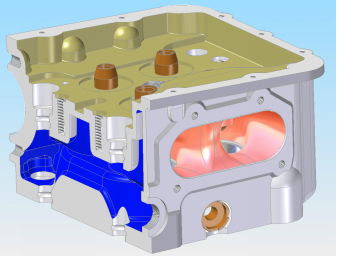Figure 5. Simplified model, exhaust valve side

## 6.png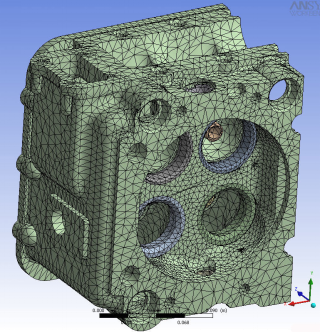Figure 6. Mesh of the simplified model

At STEP 1, the maximum interference of assembly of seats, valve guides and the maximum pre-stress of the threaded rods (studs) are considered.AT STEP 2, the thermal conditions come into play (low power load). STEP 3 adds pressure to the valve seats and to the head roof (full power load).

10. Fea Model Validation

## 7.png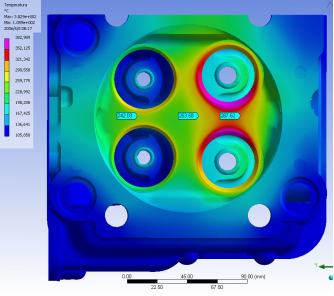Figure 7. Temperature from FEA

Table 3. Single head heat balance (W)

 Input [W] Output [W] Combustion Chamber 8562 Coolant 24715 Exhaust 13417 Intake Duct 1089 Exhaust valves seats 1418 Air conv. 20 Intake valves seats 1150 Valve guides 1082 Lubricant 29 Spark plugs 142 Total 25800 Total 25800

The validity of a model should always be verified through experimental tests when available.

Temperatures obtained by thermocouples at different points of the head are available, as well as temperature measurements and refrigerant flow through the heat exchanger from NACA TN 2069. The experimental results are summarized by NACA in readable charts. The thermal verification, then, concerns the FEA model reliability. First, you can check the power removed from the refrigerant. It can be seen from the graphs of NACA that in a Merlin engine developing 1,111 kW (1,490 HP) on the propeller shaft, the coolant subtracts about 360 BTU/s, corresponding to 380 kW of thermal power.

Please note that the coolant in the Merlin engine follows the following path:  at first it cools the cylinder liners and then goes into the head through fourteen connecting sleeves.

Therefore, the 380 kW include also the portion of the heat removed on the cylinder liners.

The total amount of heat extracted from the refrigerant, referring to a single cylinder, derived from our simulation with FEA, is 24.7 kW. This value must be multiplied by the number of cylinders (12) achieving about 300 kW overall. This value seems to be acceptable.

For the global heat balance see Tables 3 and 4.

As it can be seen the contribution of lubricant and spark plugs to the cooling is negligible. Therefore, a proper way to calculate it has not been described in this paper for sake of simplicity.

Table 4. Single head heat balance (%)

 Input [%] Output [%] Combustion Chamber 33.19 Coolant 95.7 Exhaust duct 52 Intake Duct 4.3 Exhaust valves seats 5.5 Air conv. - Intake valves seats 4.2 Valve guides 4.2 Lubricant 0.1 Spark plugs 0.5 Total 100 Total 100

As it can be seen a large part of the input energy comes from the exhaust duct. The second significant contribution is given by the combustion chamber. The other contributions are negligible in engineering terms.

A comparison between the temperatures measured by NACA and the results from FEA show an extremely good correlation (Table 5) and Figures 7, 8 and 9.

Table 5. Comparison between experimental and simulated results

 T NACA [K] T FEA [K] Between exhaust valves 560 561 Centerline 525 527 Between intake valves 516 515

However, this is not a very good result, since NACA measured the temperatures under the skin with quite large thermocouples. The results were compensated by NACA engineers, but successive measurements made by the Authors proved that the original measured values were underestimated.

## 8.pngFigure 8. Results from FEA

Global stresses from step 3 proved to be extremely high (Figure 10). This is not a surprise, since the combustion load are instantaneous and safety factors close to unitary are common.

## 9.png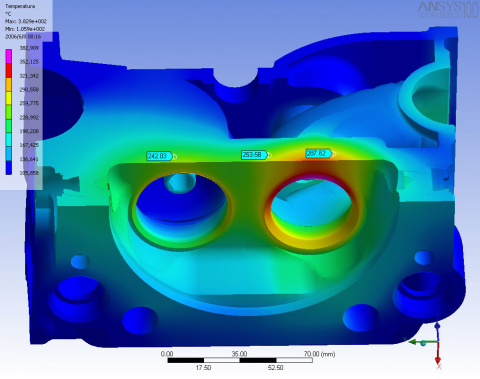Figure 9. Results from FEA

Table 4 summarizes the maximum stresses simulated by FEA on Merlin XX head. These stresses are extremely high and will be discussed in another, more detailed, paper. However, it can be pointed out that, except the first one in the hole of the stud on exhaust side, the values found are quite normal for an aluminum alloy head. Interference of valve seats and guide is important, especially when, as in this case, the maximum interference value is considered. In most cases, these values are not reached. However, since they are reported on the drawings, this worst case should be included in design simulations and evaluations.

Table 4. Maximum stresses on head

 Position σmax, [MPa] σp0.2, [MPa] T [K] Step 3 Hole of stud exhaust side 242 240 417 Step 1,2 exhaust valve seat 651 800 653 Step 1,2 Intake valve seat 180 800 578 Step 1,2 Intake valve guide 78 540 436 Step 1,2 Exhaust valve guide 138 540 470

## 10.png11. Conclusions

Some considerations on the examined component emerge from this study. The most important stresses for the head do not come from operating pressures but are of thermal nature.

It was found that the wall temperature between the exhaust valves are extremely high, around 560 K. Aluminum alloys, beyond the 473 K threshold, undergoes a remarkable decay of mechanical properties, as the effects of aging are eliminated. It's a common problem for all engine heads, but in this case, this is even more evident.

The discrepancies between the hardness values at different points of the head suggest that the material is quite heterogeneous.

Probably the differences in cooling speed in the sand casts are appreciable and this affects the structure and size of the grain.

This shortcoming could be only partially corrected by the following heat treatments

A modern alloy of silicon aluminum like G-Al Si 7 or G-Al Si 9, guarantees better quality castings.

Today's casting technology enables the solidification of the eutectic aluminum-silicon phase into the globular, thus increasing the alloy resistance characteristics.

It is emphasized that, at present, there is an orientation towards the use of aluminum-copper alloys with far superior mechanical characteristics. However, this advantage is paid with a worse resistance to corrosion.

From the simulation then emerged the problem of wall temperature, between the exhaust ducts. It is excessive, as it goes beyond 433 K. Certainly the tight space between the ducts does not help heat exchange, the liquid tends to form stagnant pockets, as evidenced by the deposits visible on the coolant ducts themselves. Even if the head is redesigned, the margins of intervention are rather small, as they are bound to the original outer geometric shapes. You could try to increase the liquid passage speed by increasing coolant pump pressure.

Material behavior depends on average peak temperature, stress magnitude and stress gradient. This assumption is valid since temperatures varies slowly when compared to pressure (stress). In this paper, a RR Merlin head is simulated with a few mathematical models used in Formula 1 racing. These extremely simplified model makes it possible to evaluate temperatures and pressures starting from very few data. The method is described in detail, along with the many experimental coefficients available from several years of design activity. A step by step approach is used to allow the comprehension of this method that was developed by the Authors. The choice of the RR Merlin was dictated by the public availability of experimental data on temperatures. In fact, in the case of the RR Merlin XX very reliable experimental results are available on a NACA TN 2069. A reverse engineering process was applied on a rescued RR Merlin XX head. An accurate redesign was performed to obtain a 3D model. Assembly instructions and tolerances were found on Rolls Royce overhaul manuals. In this way assembly and working loads were calculated and simulated. Nonlinear FEA analysis was performed on this CAD model with extremely satisfactory results for the thermal loads. Well known criticalities of the original design were found. The results were compared with NACA results both for heat rejection and temperatures.

References

 Piancastelli L., Frizziero L., Morganti E., Canaparo A. (2012). Fuzzy control system for aircraft diesel engines, International Journal of Heat and Technology, ISSN 0392-8764, Vol. 30, No. 1, pp. 131-135.

 Piancastelli L., Frizziero L. Rocchi I. (2012). Feasible optimum design of a turbo compound Diesel Brayton cycle for diesel-turbo-fan aircraft propulsion, International Journal of Heat and Technology, Vol. 30, No. 2, pp. 121-126.

 Piancastelli L., Frizziero L., Daidzic N.E., Rocchi I. (2016). Analysis of automotive diesel conversions with KERS for future aerospace applications, International Journal of Heat and Technology, Vol. 31, No. 1.

 Piancastelli L., Frizziero L., Morganti E., Pezzuti E. (2012). Method for evaluating the durability of aircraft piston engines, Walailak Journal of Science and Technology, Vol. 9, No. 4, pp. 425-431.

 Piancastelli L., Cassani S. (2017). Maximum peak pressure evaluation of an automotive common rail diesel piston engine head, ARPN Journal of Engineering and Applied Sciences, Vol. 12, pp. 212-218.